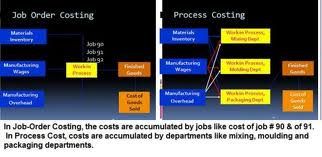Accounting

# What is the Mean by Process Costing?What is the Mean by Process Costing?

Process costing is a term used in cost accounting to describe one method for  collecting and assigning manufacturing costs to the units produced. Processing cost is used when nearly identical units are mass produced. (Job costing or job order costing is a method used when the units manufactured vary significantly from one another.

To illustrate process costing, let’s assume that a product requires several processing operations—each of which occurs in a separate department. The costs of Department One for the month of June amount to \$150,000 of direct materials and \$225,000 of conversion costs (direct labor and manufacturing overhead). If the number of units processed in June in Department One is the equivalent of 100,000 units, the per unit cost of the products processed in Department One in June will be \$1.50 for direct materials and \$2.25 for conversion costs. These costs will then be transferred to Department Two and its processing costs will be added to the cost of the units. It involves the following steps:

(1) the ‘total cost per process’ is computed by estimating the number of products passing through each process in a given period.

(2) the ‘unit cost per process’ is computed by dividing the ‘total cost per process’ by the number of units passing through the process in the given period.

(3) the ‘unit cost per process’ is charged to each unit as it passes through each process so that, at the end of the production cycle, each product will have received an appropriate charge for each process through which it has passed.